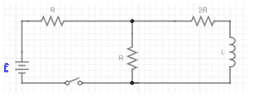# Can't find total resistance in a complex star circuit

Chrys
[Thread moved from the technical forums to the schoolwork forums by the Mentors]

Hi i have this assignment for homework:There is only one battery for the circuit, E=10V, R=4 Ohms and L=1H
it asks me to find the time constant of the circuit. i know that a time constant in a RL circuit is t=L/R
but i can't find the Rtotal since its in a star direction.

Thanks!

Last edited by a moderator:
•Delta2

Gold Member
2022 Award
So you have to analyze the circuit completely. So you should start to write down the differential equations for the relevant voltages and currents!

Chrys
what do you mean? i am kind of bad at physics
and in my university we just started learning integrals

•Delta2
alan123hk
There are basically two ways to solve this problem.

1. formal and rigorous approach
Write the system of differential equations for this circuit using Kirchhoff's laws, then simplify the differential equations to find the time constant

2. Fast methods commonly used in engineering
Use Thevenin's theorem to quickly find the equivalent series resistance, and then directly write the time constant.

•Delta2, hutchphd, DaveE and 1 other person
Homework Helper
Gold Member
2022 Award
Hi i have this assignment for homework:
Hi @Chrys. Welcome to PF. Your question might be better in the 'Introductory Physics Homework Help' section. Maybe one of the mentors will move it there.

The rules here require that you show evidence of your own effort before we offer guidance. See https://www.physicsforums.com/threads/homework-help-guidelines-for-students-and-helpers.686781/

However, to help you get started, I will just say two words: Thevenin’s Theorem!

Edit. Aha! @alan123hk beat me to it by a few nanoseconds!

•berkeman
alan123hk
beat me to it by a few nanoseconds!
I'm sorry for that, I didn't mean to

•Delta2 and Steve4Physics
Mentor
I'm sorry for that, I didn't mean to

Chrys
and in my circuit what is my load resistor?

Homework Helper
Gold Member
2022 Award
and in my circuit what is my load resistor?
Treat the inductor as the (Thevenin) load. Show us your working and we will look it over.

(Thevenin's theorem isn't just limited to resistors. Do a bit of background reading/research for yourself - you are at university level now!)

Chrys
•Steve4Physics
Homework Helper
Gold Member
2022 Award
I've reached till here but i don't know how to continue...
You have correctly calculated the Thevenin voltage as ##V_{Th} = 5V##. (If you used the voltage (potential) divider formula, you could have done it without first finding the current.)

However, you don’t actually need ##V_{Th}## to answer this question!

And note that in your final diagram, you should show the (Thevenin) supply voltage as 5V, not 10V.

You have correctly found the Thevenin resistance to be ##R_{Th} = 10Ω##.

***To find the time constant, you now pretend the circuit is simply a battery, 10Ω resistor and 1H inductor in series.***

What is the standard formula for the time constant of an RL series circuit?

•hutchphd
Chrys
time constant in a RL circuit is t=R/L, in my final circuit the battery should be 5V right?

Homework Helper
Gold Member
2022 Award
time constant in a RL circuit is t=R/L, in my final circuit the battery should be 5V right?
Yes. Your Thevenin equivalent circuit is a 5V supply, a 10Ω resistor and a 1H inductor all in series. But the voltage is not needed if all you want to do is to find the time constant.

So the time constant is ?

Chrys
0,1s. Later on in the excersice it asks me to find something else with time=10 time constant, it should be 1s right?

•Steve4Physics
Homework Helper
Gold Member
2022 Award
0,1s. Later on in the excersice it asks me to find something else with time=10 time constant, it should be 1s right?
Yes and yes.

It's good practice to show all working clearly:
##\tau = \frac L {R_{Th}} = \frac {1H} {10\Omega} = 0.1s##
(Here in the UK we use a dot to represent the decimal position.)

0,1s. Later on in the excersice it asks me to find something else with time=10 time constant, it should be 1s right?
In my opinion the constant has to be -0.1 s.

alan123hk
In my opinion the constant has to be -0.1 s.
Do you mean the time constant should be negative?
Can you explain further?

I remember in my previous study and work experience that when we referred to the time constant by itself, we simply represented it as a positive number (L/R)).

Chrys
i think that using basic power properties its 1/(e^(10*t)) and we write e^(-10*t) because its more simple to the eye. Also how would you explain and use negative time?!?

•Homework Helper
Gold Member
2022 Award
 If Ic=0.5*(1-e^(-10*t)) then the time constant it is 1/-10=-0.1​
If ##\tau## is the time constant, the exponential decay factor is written as:
##e^{- \frac t {\tau}}##

This is the ‘standard format’, the minus sign is always present.
Both time (##t##) and the time constant (##\tau##) are positive quantities.

For example if we write:
##e^{- \frac t {0.1}}##
we are using ##\tau= 0.1## not ##\tau= -0.1##.

••